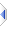# factorial

 Definition: atom a = factorial(integer n) Description: Standard iterative factorial function, with memoisation. Comments: Returns inf for n>170 (1754 on 64-bit). Atoms on 32-bit are limited to 53 bits of precision, which means that the largest factorial which can be held exactly is 18 (20 on 64-bit), above that up to the limits above are approximations. There is also a bigatom version, ba_factorial(), with more accuracy and higher limits, which is a modified copy of this routine that likewise demands an integer argument, however I cannot state anything particularly useful about the extended accuracy or limits that provides, beyond noting that the memoised results are held in a normal sequence, which is calculated sequentially, so to obtain ba_factorial(10,000) it must invoke or have invoked ba_mul() at least 10,000 times, with corresponding performance implications. Ancilliary functions: atom res = k_perm(integer n, k) -- standard partial permutations calculation (sequences without repetition) atom res = choose(integer n, k) -- standard combinations calculation - choose k from n aka "n choose k" The k_perm routine calculates the result directly, rather than via the slightly less efficient (/limit-exceeding) way of using the complete factorials, ie n(n-1)..(n-k-1) rather than n!/(n-k)! and the choose routine simply divides that by factorial(k). Implementation: See builtins\factorial.e (an autoinclude) for details of the actual implementation. Example: ```-- n : 0 1 2 3 4 5 6 7 8 -- factorial(n): 1 1 2 6 24 120 720 5040 40320 ```